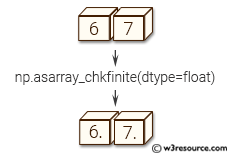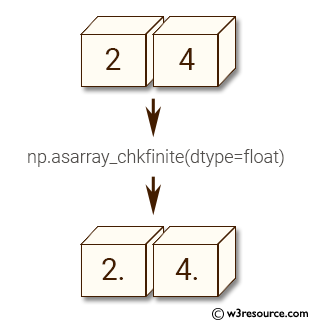# NumPy Array manipulation: asarray_chkfinite() function

## numpy.asarray_chkfinite() function

The asarray_chkfinite() function is used to convert the input to an array, checking for NaNs or Infs.

Syntax:

`numpy.asarray_chkfinite(a, dtype=None, order=None)`Version: 1.15.0

Parameter:

Name Description Required /
Optional
a Input data, in any form that can be converted to an array. This includes lists, lists of tuples, tuples, tuples of tuples, tuples of lists and ndarrays. Success requires no NaNs or Infs. Required
dtype By default, the data-type is inferred from the input data. Optional

Return value:

out : ndarray - Array interpretation of a. No copy is performed if the input is already an ndarray. If a is a subclass of ndarray, a base class ndarray is returned.

Example-1: numpy.asarray_chkfinite()

``````>>> import numpy as np
>>> a = [2, 4]
>>> np.asarray_chkfinite(a, dtype=float)
array([ 2.,  4.])
``````

Pictorial Presentation:Example-2: Raises asarray_chkfinite

``````>>> import numpy as np
>>> a = [2, 4, np.inf]
>>> try:
...   np.asarray_chkfinite(a)
... except ValueError:
...   print('ValueError')
...
ValueError
``````

Python - NumPy Code Editor:

Previous: ascontiguousarray
Next: asscalar()

﻿